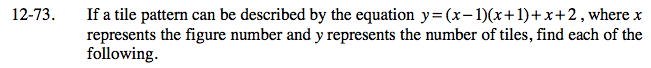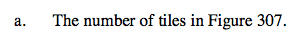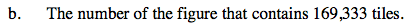### Home > AC > Chapter 12 > Lesson 12.4.2 > Problem12-73

12-73.Substitute 307 into the equation for x.

y = (307 − 1)(307 + 1) + 307 + 2

Solve for y.

y = 94,557Set y = 169,333.

169,333 = (x − 1)(x + 1) + x + 2

Solve for x.